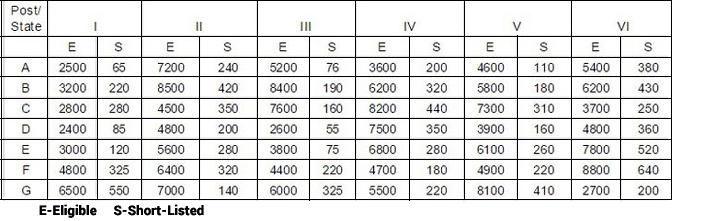## SBI Clerk DA Test 1

Instructions

Study the following table carefully and answer the questions which follow-

Number of candidates found Eligible and the number of candidates Short-listed for interviews for a recent Recruitment process for Six posts from Different States.Question 1

From State ‘B’ which post had the ‘’highest’’ percentage of candidates short-listed?

Question 2

‘’Approximately’’ what is the average number of candidates found eligible from all States for Post III?

Question 3

What is the overall percentage (rounded off to one digit after decimal)of candidates short-listed over the total number of candidates eligible from all the States together for Post I?

Question 4

What is the ratio between total number of candidates short-listed for all the posts together from States E and G respectively?

Question 5

Total number of candidates found eligible from all States together for Post I is ‘’approximately’’ what percent of the total number of candidates found eligible from all States together for Post VI?